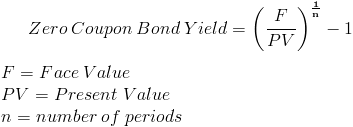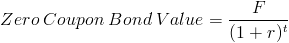# Zero Coupon Bond Effective YieldThe zero coupon bond effective yield formula is used to calculate the periodic return for a zero coupon bond, or sometimes referred to as a discount bond.

A zero coupon bond is a bond that does not pay dividends (coupons) per period, but instead is sold at a discount from the face value. For example, an investor purchases one of these bonds at \$500, which has a face value at maturity of \$1,000. Although no coupons are paid periodically, the investor will receive the return upon maturity or upon sell assuming that the rates remain constant.

## Zero Coupon Bond Effective Yield Formula vs. BEY Formula

The zero coupon bond effective yield formula shown up top takes into consideration the effect of compounding. For example, suppose that a discount bond has five years until maturity. If the number of years is used for n, then the annual yield is calculated. Considering that multiple years are involved, calculating a rate that takes time value of money and compounding into consideration is needed. An investment that pays 10% per year is not equivalent to a 10 year discount bond that pays a 100% return after ten years. The investment that pays 10% can be reinvested and by compounding the returns(or considering the time value of money), the total return after 10 years would bewhich would equal 259%.

In contrast, the formula for the bond equivalent yield does not take compounding into consideration. For this reason, the formula for bond equivalent yield is primarily used to compare discount bonds of short maturity, specifically less than one year.

## How is the Zero Coupon Bond Effective Yield Formula Derived?

The formula for calculating the effective yield on a discount bond, or zero coupon bond, can be found by rearranging the present value of a zero coupon bond formula:This formula can be written asThis formula will then becomeBy subtracting 1 from the both sides, the result would be the formula shown at the top of the page.

New to Finance?